# Flux of a vector field

(diff) ← Older revision | Latest revision (diff) | Newer revision → (diff)

A concept in the theory of vector fields. The flux of a vector fieldthrough the surface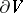is expressed, up to sign, by the surface integral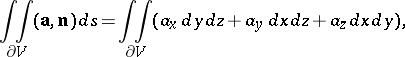whereis the unit normal vector to the surface(it is assumed that the vectorchanges continuously over the surface). The flux of the velocity field of a fluid is equal to the volume of fluid passing through the surfaceper unit time.

The flux of a differential vector field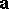(defined by the formula above) is related to the divergence of: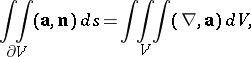where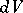is the volume element inandis the Hamilton operator,. This equation is called the divergence theorem or also Green's theorem in space, cf. [a1] and Stokes theorem.